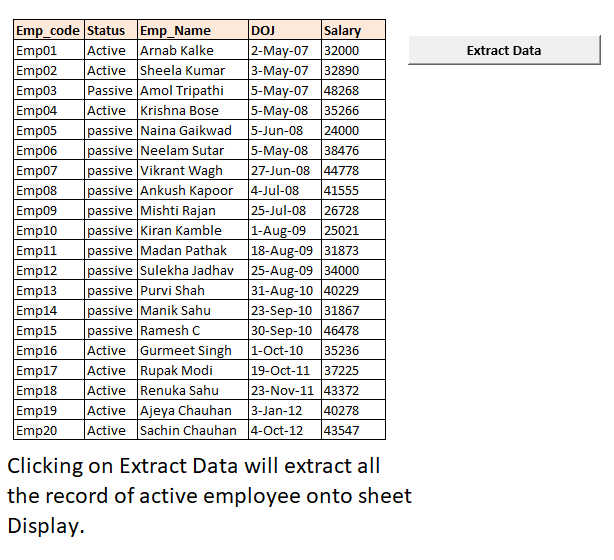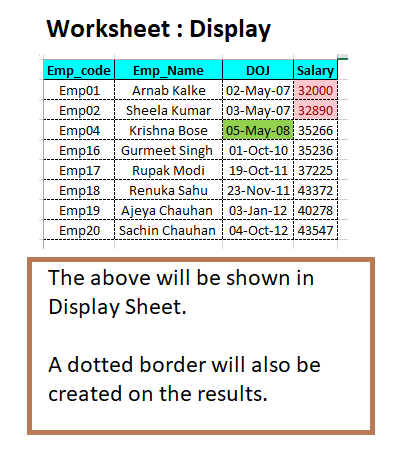# Excel Macros Series 1: Extract Active Employee Data & conditionally format extracted records

In our below example we have a data of employee records.

Our requirement.

From our worksheet emp_master datadet  we first find out the active employee data and then copy the active employee records onto worksheet display.Then in our extracted records below conditional formatting is set.

• In column DOJ all employee whose have joined in 2008 are highlighted.
• In column Salary all employee whose have salary less than Rs 33000/- are highlighted.

Note : How to use Macros (Know More)```
Sub extract_emp_data()
Dim counttotrows As Integer
Dim status As String
Dim loopcounter As Integer
Dim actempcode As String

wsdisplay.Activate
Dim del_old_records As Range

Set del_old_records = Range("A2", Range("A2").End(xlDown).End(xlToRight))
del_old_records.Delete
Range("A2").Select

wsemp.Activate
counttotrows = Application.WorksheetFunction.CountA(Range("B3", Range("B" & Rows.Count).End(xlUp)))
Range("B3").Select
loopcounter = 0

Do
actempcode = Selection.Value
status = Selection.Offset(0, 1).Value

If status = "Active" Then
loopcounter = loopcounter + 1
wsdisplay.Activate
ActiveCell.Value = actempcode
ActiveCell.Offset(0, 1).Value = Application.WorksheetFunction.VLookup(ActiveCell.Value, [empmaster], 3, False)
ActiveCell.Offset(0, 2).Value = Application.WorksheetFunction.VLookup(ActiveCell.Value, [empmaster], 4, False)
ActiveCell.Offset(0, 3).Value = Application.WorksheetFunction.VLookup(ActiveCell.Value, [empmaster], 5, False)
ActiveCell.Offset(1, 0).Select
wsemp.Activate
ActiveCell.Offset(1, 0).Select
Else
loopcounter = loopcounter + 1
wsemp.Activate
ActiveCell.Offset(1, 0).Select
End If
Loop While loopcounter <> counttotrows

' Set conditional formatting
wsdisplay.Activate
Dim cf_range As Range
Set cf_range = Range("D2", Range("D2").End(xlDown))
cf_range.Select

'' Specify Parameter
Selection.FormatConditions(Selection.FormatConditions.Count).SetFirstPriority

With Selection.FormatConditions(1).Font
.Color = -16383844
End With
With Selection.FormatConditions(1).Interior
.PatternColorIndex = xlAutomatic
.Color = 13551615
End With
Selection.FormatConditions(1).StopIfTrue = False

Dim cf_range2 As Range
Set cf_range2 = Range("C2", Range("C2").End(xlDown))
cf_range2.Select
Selection.FormatConditions(Selection.FormatConditions.Count).SetFirstPriority
With Selection.FormatConditions(1).Interior
.PatternColorIndex = xlAutomatic
.Color = 5296274
End With
Selection.FormatConditions(1).StopIfTrue = False

Range("A1:D1").Interior.Color = vbCyan
Range("A1:D1").Font.Bold = True
Range("A2").CurrentRegion.EntireColumn.AutoFit
Range("A2", Range("A2").End(xlDown).End(xlToRight)).HorizontalAlignment = xlCenter
Range("A2", Range("A2").End(xlDown).End(xlToRight)).Borders.LineStyle = xlDash
Range("A2", Range("A2").End(xlDown).End(xlToRight)).Borders.Weight = xlThin
Range("C2", Range("C2").End(xlDown)).NumberFormat = "dd-mmm-yy;@"
Range("D2", Range("D2").End(xlDown)).NumberFormat = "0"

wsemp.Activate
Range("B3").Select
wsdisplay.Activate
Range("A2").Select

MsgBox ("Active employee data extraction completed")

End Sub

```